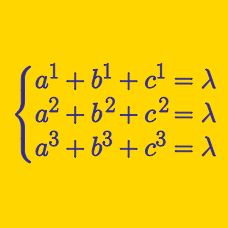Algebra

# Symmetric Polynomials: Level 5 Challenges

If $a,b \ \& \ c$ are the roots of the polynomial $x^{3}-2x^{2}+3x+1$

Find the value of $a^{5}+b^{5}+c^{5}-(a^{4}+b^{4}+c^{4})$

If $V_{n}=a^{n}+b^{n},$ where $a$ and $b$ are the roots of $x^{2}+x+1,$ what is the value of

$\sum_{n=0}^{1729} (-1)^{n} \cdot \ V_{n} ?$

Find the value of $-a$ for which the roots $x_{1}, x_{2}, x_{3}$ of $x^{ 3 }-6x^{2}+ax-a = 0$ satisfy $\left( x_{1}-3 \right)^{3}+\left(x_{2}-3 \right)^{3}+\left(x_{3}-3 \right)^{ 3 } = 0$.

$\Large{P(x) = x^3 - 3x+1}$

Let $Q(x) = x^3 + Ax^2 + Bx + C$ be a polynomial with integer coefficients such that its roots are the fifth powers of the roots of $P(x)$. Evaluate $A+B+C$.

$\large \begin{cases} {a+b+c=9} \\ {a^2+b^2+c^2=99} \\ {a^3+b^3+c^3 = 999} \end{cases}$

If $a,b$ and $c$ are complex numbers that satisfy the system of equations above, find the remainder of $a^5+b^5+c^5$ when divided by 78.

×